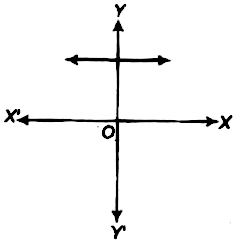Home/Class 10 Math Chapter List/2. Polynomials/

# The graph of (y=p(x)) is given in figure below, for some polynomial (p(x)). Find the number of zeroes of (p(x)).

## QuestionMathClass 10

The graph of $$y=p(x)$$ is given in figure below, for some polynomial $$p(x)$$. Find the number of zeroes of $$p(x)$$.Number of  zeroes is zero.
4.64.6## Solution

Using graph of a polynomial we can find the number of zeroes of a polynomial by looking how many times the graph of $$p(x)$$  cuts or touches the $$x$$-axis.
As the graph of $$p(x)$$ does not touch or intersect$$x$$-axis.
Therefore, There are no zeroes of $$p(x)$$ .